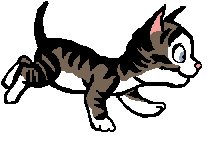# Math GamesCounting and Number Identification

Counting Games            Various Counting Games within 10 and 20

Word Problems

Base Ten Bingo                     Place Value ones/tens/hundreds

Base Ten Fun                         Place Value ones/tens/hundreds

Place Value                              Counting Groups of Ten (need to register for free after 2 games)

Balloon Subtraction        Subtraction Problems

Subtraction Problems

Subtraction Problems

Subtraction Problems

Various Math Games

Various Math Games

Various Math Games

Various Math Games